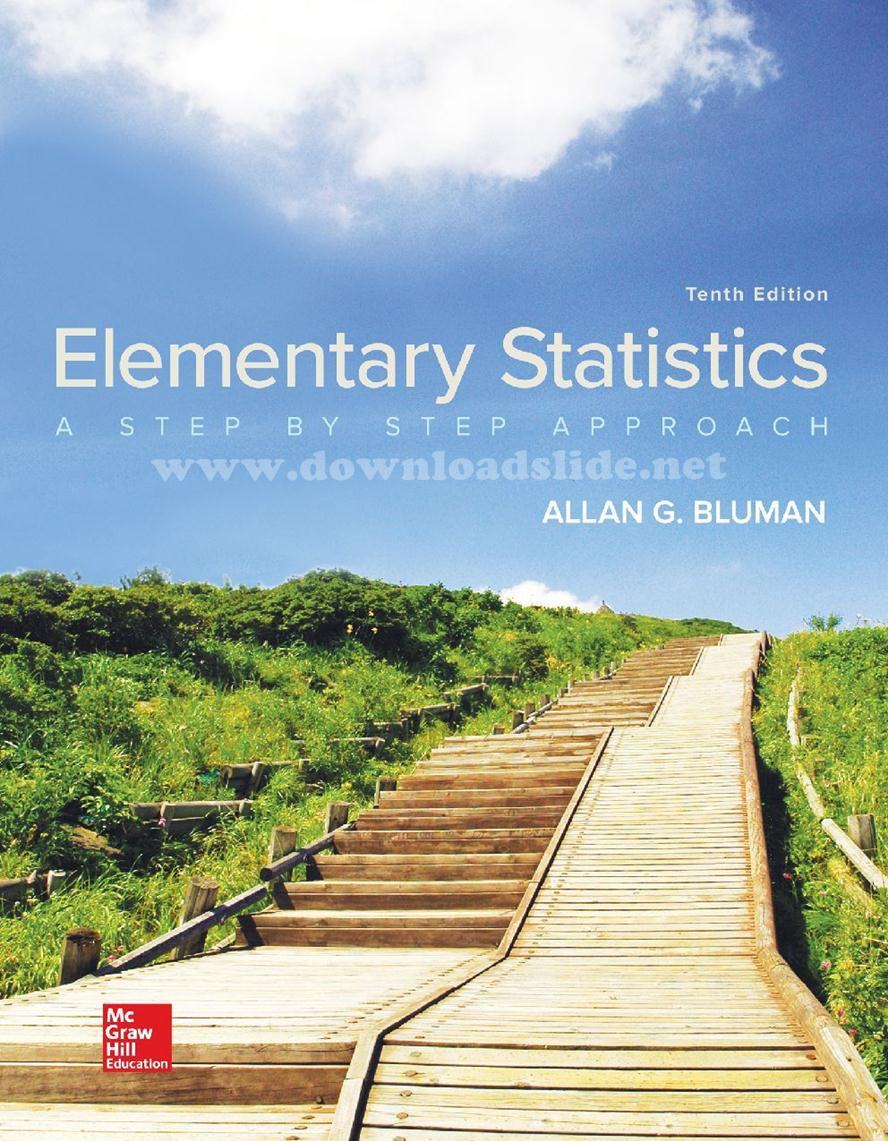### Ebook Elementary Statistics 10e by BluemanElementary Statistics: A Step By Step Approach
10th Edition
by Allan Bluman
ISBN13: 9781259755330

Table of Content:
1 The Nature of Probability and Statistics
2 Frequency Distributions and Graphs
3 Data Description
4 Probability and Counting Rules
5 Discrete Probability Distributions
6 The Normal Distribution
7 Confidence Intervals and Sample Size
8 Hypothesis Testing
9 Testing the Difference Between Two Means, Two Variances, and Two Proportionswo Variances
10 Correlation and Regression
11 Other Chi-Square Tests
12 Analysis of Variance
13 Nonparametric Statistics
14 Sampling and Simulation